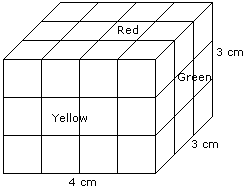# Verbal Reasoning - Cube and Cuboid - Discussion

The following questions are based on the information given below:

1. There is a cuboid whose dimensions are 4 x 3 x 3 cm.
2. The opposite faces of dimensions 4 x 3 are coloured yellow.
3. The opposite faces of other dimensions 4 x 3 are coloured red.
4. The opposite faces of dimensions 3 x 3 are coloured green.
5. Now the cuboid is cut into small cubes of side 1 cm.

3.

How many small cubes will have no face coloured ?

 [A]. 1 [B]. 2 [C]. 4 [D]. 8

Explanation:Number of small cubes have no face coloured = (4 - 2) x (3 - 2) = 2 x 1 = 2

 Mano said: (Apr 28, 2011) How we can find it as (4 - 2) x (3 - 2) ?

 Sakthi said: (Aug 25, 2011) To find the faces that have not been colored can be given by the formula(x-2)^3, for a cube l, b, h are same so can directy use, whereas for cuboid. Since they ve different dimensions, its given as (l-2) *(b-2)*(h-2) here l=4 other values are 3, So, 2*1*1 =2

 Rakeshsoni said: (Oct 2, 2017) Can anyone explain this?

 Pinky said: (Aug 10, 2018) Total cubes = 4*3*3 = 36. Cubes with 3 faces coloured = 8. Cubes with 2 faces coloured = 16 (6+6+2+2). Cubes with 1 face colured = 10 (2+2+1+1+2+2). So, cubes with no coloured face = 36-(8+16+10) = 36-34 = 2.